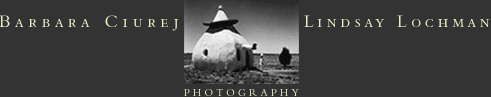A R C H I T E C T U R EP O R T F O L I O S:N E W   S T O N E   A G EC O R P O R A T E   C O R R I D O R ST H E   L A S T   S T A N DP R I M E   L O C A T I O N SA G E   O F   T H E   D O M I C I L E Shome  l  artists’ statement  l  contact us